CBSE Sample Papers for Class 12 Physics Paper 3 are part of CBSE Sample Papers for Class 12 Physics. Here we have given CBSE Sample Papers for Class 12 Physics Paper 3.

## CBSE Sample Papers for Class 12 Physics Paper 3

 Board CBSE Class XII Subject Physics Sample Paper Set Paper 3 Category CBSE Sample Papers
Students who are going to appear for CBSE Class 12 Examinations are advised to practice the CBSE sample papers given here which is designed as per the latest Syllabus and marking scheme as prescribed by the CBSE is given here. Paper 3 of Solved CBSE Sample Paper for Class 12 Physics is given below with free PDF download solutions.
Time Allowed : 3 Hours
Max. Marks : 70
General Instructions
• All questions are compulsory. There are 26 questions in all.
• This question paper has five sections: Section A, Section B, Section C, Section D and Section E.
• Section A contains five questions of 1 mark each. Section B contains five questions of 2 marks each. Section C contains twelve questions of 3 marks each. Section D contains one value based question of 4 marks and Section E contains three questions of 5 marks each.
• There is no overall choice. However, an internal choice has been provided in 1 question of 2 marks, 1 question of 3 marks and all the 3 questions of 5 marks weightage. You have to attempt only 1 of the choices in such questions.
• You may use the following values of physical constants wherever necessary :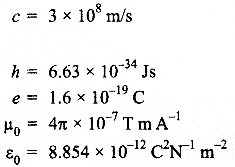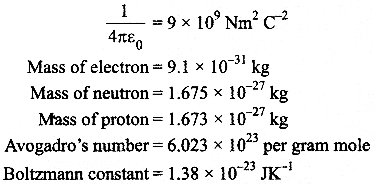Questions :
SECTION : A

Question 1.
In which orientation, a dipole placed in a uniform electric field is in
(i) stable,
(ii) unstable equilibrium?

Question 2.
Which part of electromagnetic spectrum has largest penetrating power?

Question 3.
A plot of magnetic flux (Φ) versus current (I) is shown in the figure  for two inductors A and B. Which of the two has larger value of self inductance.

Question 4.
Figure shows three point charges, + 2q, -q and +3q. Two charges + 2q and -q are enclosed within a surface ‘S’. What is the electric flux due to this configuration through the surface ‘S’?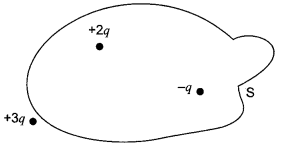Question 5.
A glass lens of refractive index 1.45 disappears  when immersed in a liquid. What is the value of refractive index of the liquid.

SECTION : B

Question 6.
What is the ratio of radii of the orbits corresponding to first excited state and ground state in a hydrogen atom?

Question 7.
A wire of resistance 8R,is bent in the form of a circle. What is the effective resistance between the ends of a diameter AB?

Question 8.
State the conditions for the phenomenon of total internal reflection to occur.

Question 9.
Explain the function of a repeater in a communication system.

Question 10.
(i) Write two characteristics of a material used for making permanent magnets.
(ii) Why is core of an electromagnet made of ferromagnetic materials?

OR

Draw magnetic field lines when a
(i) diamagnetic,
(ii) paramagnetic substance is placed in an external magnetic field. Which magnetic property distinguishes this behaviour of the field lines due to the two substances?

SECTION : C

Question 11.
Draw a circuit diagram of an illuminated photodiode in reverse bias. How is photodiode used to measure light intensity?

Question 12.
An electric lamp having coil of negligible inductance connected in series with a capacitor and an AC source is glowing with certain brightness. How does the brightness of the lamp change on reducing the
(i) capacitance, and

Question 13.
Arrange the following electromagnetic radiations in ascending order of their frequencies :

1. Microwave
3. X-rays
4. Gamma rays

Write two uses of any one of these.

Question 14.
The radii of curvature of the faces of a double convex lens are 10 cm and 15 cm. If focal length of the lens is 12 cm, find the refractive index of the material of the lens.

Question 15.
An electron is accelerated through a potential difference of 100 volts. What is the de-Broglie wavelength associated with it? To which part of the electromagnetic spectrum does this value of wavelength correspond?

Question 16.
A heavy nucleus X of mass number 240 and binding energy per nucleon 7.6 MeV is split into two fragments Y and Z of mass numbers 110 and 130. The binding energy of nucleons in Y and Z is 8.5 MeV per nucleon. Calculate the energy Q released per fission in MeV.

Question 17.

1. The bluish colour predominates in clear sky.
2. Violet colour is seen at the bottom of the spectrum when white light is dispersed by a prism. State reasons to explain these observations.

Question 18.
Plot a graph showing the variation of stopping potential with the frequency of incident radiation for two different photosensitive materials having work functions W1 and W2 (W1 > W2).
On what factors does the
(i) slope and
(ii) intercept of the lines depend?

Question 19.
A parallel plate capacitor is charged by a battery. After sometime the battery is disconnected and a dielectric slab with its thickness equal to the plate separation is inserted between the plates. How will
(i) the capacitance of the capacitor,
(ii) potential difference between the plates and
(iii) the energy stored in the capacitor be affected?

Question 20.
Write the principle of working of a potentiometer. Describe briefly, with the help of a circuit diagram, how a potentiometer is used to determine the internal resistance of a given cell.

Question 21.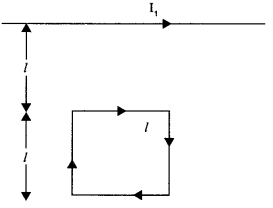Write the expression for the magnetic moment ( m ) due to a planar square loop of side l carrying a steady current I in a vector form. In the given figure this loop is placed in a horizontal plane near a long straight conductor carrying a steady current I1 at a distancel as shown.
Given reasons to explain that the loop will experience a net force but no torque. Write the expression for this force acting on the loop.

Question 22.
(a) Depict the equipotential surfaces for a system of two identical positive point charges placed at a distance d apart.
(b)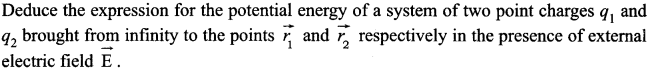SECTION : E

Question 23.
For the last four months, the English teacher of Komal had erratic body movement, lack of coordination etc in her daily activities. Occasionally she had headache also. Komal suggested to get a MRI and other complete medical check up. After that she was diagnosed a brain tumour.

1. What values are displayed by Komal?
2. How can radioisotopes help a doctor to diagnose brain tumour?

Question 24.
Describe briefly, with the help of a labelled diagram, the basic elements of an A.C. generator. State its underlying principle. Show diagrammatically how an alternating emf is generated by a loop of wire rotating in a magnetic field. Write the expression for the instantaneous value of the emf induced in the rotating loop

OR

A series LCR circuit is connected to an ac source having voltage V = Vm sin cot. Derive the expression for the instantaneous current T and its phase relationship to the applied voltage. Obtain the condition for resonance to occur. Define ‘power factor’. Sate the conditions under which it is
(i) maximum and
(ii) minimum.

Question 25.
State Huygens principle. Show, with the help of a suitable diagram, how this principle is used to obtain the diffraction pattern by a single slit. Draw a plot of intensity distribution and explain clearly why the secondary maxima become weaker with increasing order (n) of the secondary maxima.

OR

Draw a ray diagram to show the working of a compound microscope. Deduce an expression for the total magnification when the final image is formed at the near point. In a compound microscope, an object is placed at a distance of 1.5 cm from the objective of focal length 1.25 cm. If the eye-piece has a focal length of 5 cm and the final image is formed at the near point, estimate the magnifying power of the microscope.

Question 26.
(a) Explain the formation of depletion layer and potential barrier in a p-n junction output.
(b) In the figure given below the input waveform is converted into the output waveform by a device ‘X’. Name the device and draw its circuit diagram.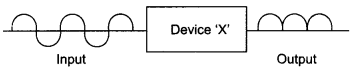(c) Identify the logic gate represented by the circuit as shown and write its truth table.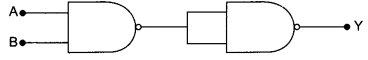OR

(a) Draw the circuit diagram of a common emitter transistor as an amplifier, define current gain and voltage gain of it.
(b) Distinguish between a conductor, a semiconductor and an insulator on the basis of energy band diagrams.

SECTION : A

(i) The dipole is in stable equilibrium when electric dipole is in the direction of electric field (θ = 0°).
(ii) The dipole is in unstable equilibrium when electric dipole is in the opposite direction of electric field (θ = 180°).

Gamma rays.

Line A.Total charge within a surface S +2q + (-q) = +q
∴ Electric flux Φ = q/ε0

The value of refractive index of the liquid is 1.45.

SECTION : B

Since r ∝ n2
For ground state, n = 1
For first state , n = 2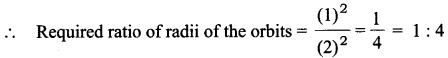Resistance of each semi-circular part of circle is 4R.
∴ R1 = R2 = 4R
Since two resistors are in parallel.
∴  Effective resistance (Re) is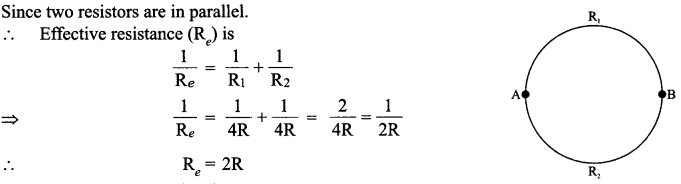(i) Light should travel from denser to rarer medium
(ii) Angle of incidence in denser medium should be greater than the critical angle for the pair of media in contact.

A repeater, picks up the signal from the transmitter, amplifies and retransmits it to the receiver sometimes with a change in carrier frequency. Repeaters are used to extend the range of a communication system.

(i) Two characteristics of a material used for making permanent magnets are:

1. High Coercivity
2. High Retentivity

(ii) Core of an electromagnet made of ferromagnetic materials because of high permeability and low retentivity.

OR

(i) Behaviour of magnetic field lines when a diamagnetic substance is placed in an external magnetic field.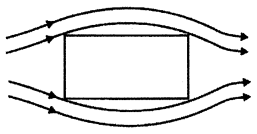(ii) Behaviour of magnetic field lines when a paramagnetic substance is placed in an external magnetic field.SECTION : C

Circuit diagram of an illuminated photodiode :Explanation :
The magnitude of the photocurrent depends on the intensity of incident light (photocurrent is proportional to incident light intensity). Thus, photodiode can be used to measure light intensity.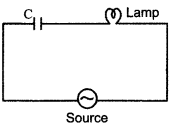When AC source is connected, the capacitor offers capacitive reactance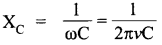The current flows in the circuit and the lamp glows.

1. On reducing the capacitance C, Xc increases. Therefore the brightness of the bulb decreases.
2. On reducing the frequency v, Xc increases. Therefore, the brightness of the bulb decrease.

Radio wave <Microwaves < X-rays <Gamma rays Uses of microwaves are :

2. Microwaves are used in microwave ovens for cooking purposes.

Here R1 = 10 cm, R2 = -15 cm,  f = 12 cm,   μ = ?
Using the lens formula,Here V = 100 volts.
The de-Broglie wavelength λ isWe have
240X = 110Y + 130Z + Energy Q
Binding energy for X = 7.6 MeV
Binding energy for two fragments Y and Z = 8.5 MeV
∴  Gain in binding energy for the nucleon = 8.5 – 7.6 = 0.9 MeV
Hence total gain in binding energy per fissioning nucleus or energy Q released per fission = 240 x 0.9 = 216 MeV

1. As per Rayleigh’s law (scattering ∝ 1/λ4), lights of shorter wavelengths scattered more by an atmospheric particles. This results in a dominance of bluish colour in the scattered light.
2. In the visible spectrum, violet light having its shortest wavelength, has the highest refractive index. Hence, it is deviated the most.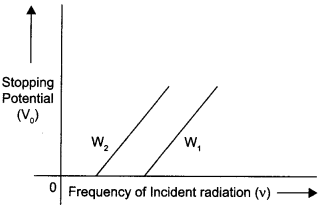The graph is showing the variation of stopping potential (V0) with the frequency of incident radiation (v0) for two different photosensitive materials having work functions W1 and W(W> W2).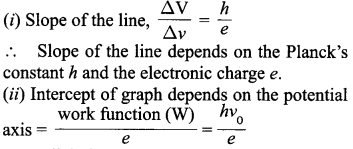As battery in disconnected, charge will remain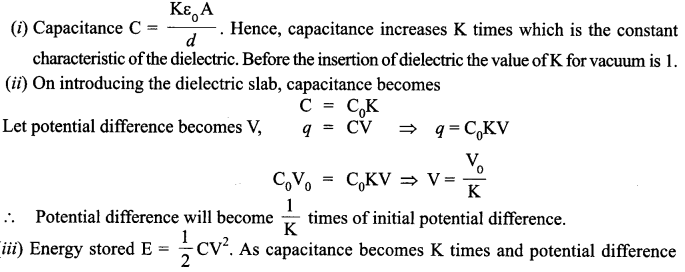Becomes 1/K times therefore, energy stored becomes 1/K times.

Working principle :
When constant current flows through a wire of uniform cross-section, then potential difference across the wire is directly proportional to the length of wire. With k2 open, balance is obtained at length l1 (AN1).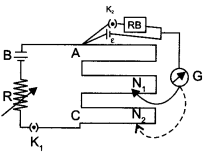Then ε = Φ (Φ = potential gradient)                                                                                     ….(1)
When key K2 is closed, the cell sends a current (I) through the resistance box (R.B.). If V is the terminal potential difference of the cell and balance is obtained at length l2 (AN2).(a) Equipotential surfaces of two identical positive point charges placed at a distance ‘d’ apart.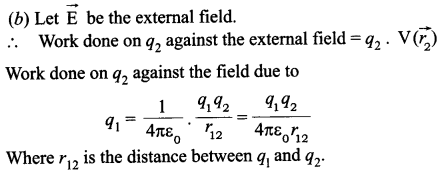By the superposition principal for fields, we add up the work done on q2 against the two fields.OR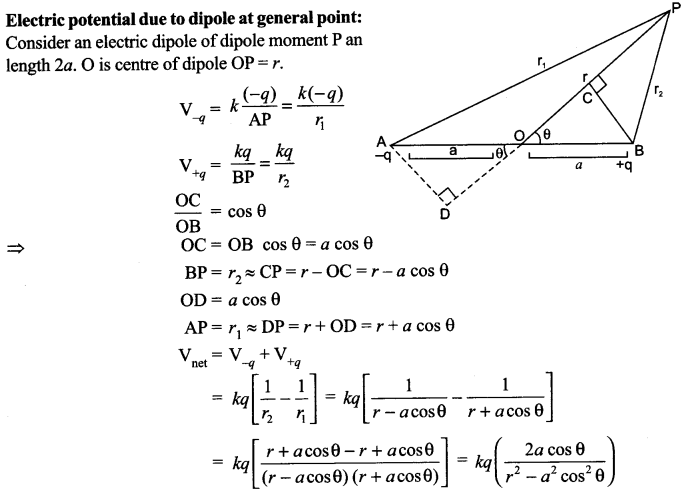SECTION : E

1. Keen observer, helpful, responsible and concerned.
2. The doctor can retrace and observe the difference between the movement of an appropriate isotope through a normal brain and a brain having tumour in it.

It consists of a coil mounted on a rotor shaft. The axis of rotation of the coil is perpendicular to the direction of the magnetic field. The axis of rotation of the coil is mechanically rotated in the uniform magnetic field by some external means. The ends of the coil are connected to an external circuit by means of slip rings and brushes.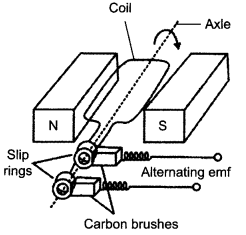Underlying Principle :
As the coil rotates in a magnetic field B, the effective area of loop (the face perpendicular to the field) which is A cos θ, where θ is the angle between area (A) and magnetic field (B) changes continuously. Hence, magnetic flux linked with the coil keeps on changing with time and an induced emf is produced.
The instantaneous value of the emf is e = NBA ω sin ωt

OR(i) Power factor is minimum when cos Φ = 0 = 1
i.e., when R = Z or XL = Xc
(ii) Power factor is minimum when cosΦ = 0
i.e., when R = 0

Huygens principle: Each point of wavefront is the source of a secondary disturbance and the wavelets emanating from these points spread out in all directions with the speed of the wave. The common tangent/forward envelope, to all these secondary wavelets gives the new wavefront at later time.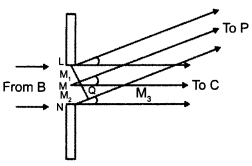Application to diffraction pattern :
All the points of incoming wavefront (parallel to the plane of slit) are in phase with plane of slit. However, the contributions of the secondary wavelets from different points, at any point, on the screen. Total contribution at any point, may add up to give a maxima or minima dependent on the phase differences.The central point is a maxima as the contribution of all secondary wavelet pairs are in phase here. Consider a next point on the screen where an angle θ = 3λ/2a. Divide the slit into three equal parts. Here the first two-thirds of the slit can be divided into two halves which have a λ/2 path difference. The contributions of these two halves cancel. Only the remaining one-third of the slit contributes to the intensity at a point between the two minima. Hence, this will be much weaker than the central maximum (where the entire slit contributes in phase). We can similarly show that there are maxima at θ = (n + 1/2) λ/a with n = 2, 3, etc. These become weaker with increasing n, since only one-fifth, one-seventh, etc. of the slit contributes in these cases.

OR

Ray diagram :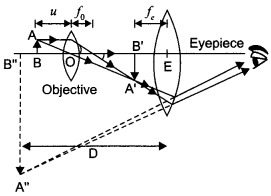Expression for total magnification :
Magnification due to the objective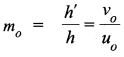Magnification me, due to eyepiece, (when the final image is formed at the near point)(a) Depletion region :
Due to the concentration gradient across p-side and n-side, holes diffuse from p-side to n-side (p → n) and electrons diffuse from n-side top-side (n → p). As the electrons diffuse from n → p, a layer of positive charge (or positive space-charge region) is developed on n-side of the junction. Similarly as the holes diffuse, a layer of negative charge (or negative space-charge region) is developed on the p-side of the junction. The space – charge region on either side of the junction together is known as depletion region.
Barrier potential :
The loss of electrons from the n-region and the gain of electron by the n-region cause a difference of potential across the junction of the two regions. The polarity of this potential is such as to oppose further flow of carriers.

(b) Full-wave rectifier :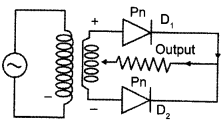(c) AND gate :OR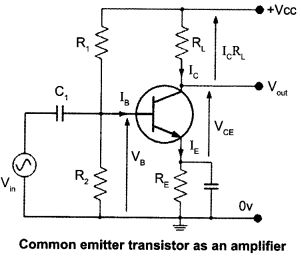(a) Common Emitter Amplifier Current and Voltage Gain :

The ratio between collector current (change in collector current) and base current (change in base current) is equal to current gain and voltage gain is given by the product of current gain and ratio of output resistance of the collector circuit to the input resistance of base circuit.(b)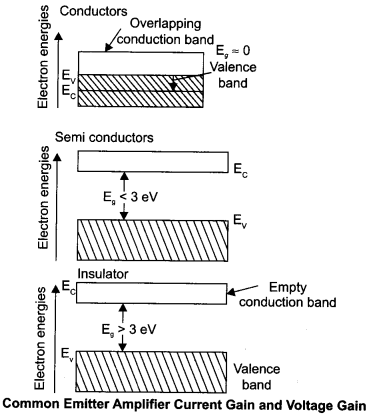We hope the CBSE Sample Papers for Class 12 Physics Paper 3 help you. If you have any query regarding CBSE Sample Papers for Class 12 Physics Paper 3, drop a comment below and we will get back to you at the earliest.